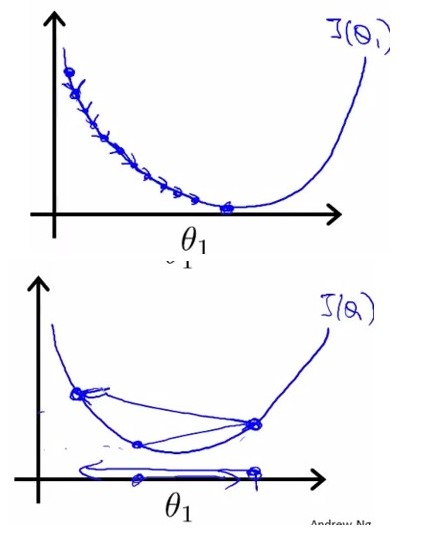# 机器学习实战ByMatlab（五）Logistic Regression

【原创】Liu_LongPo 转载请注明出处
【CSDN】http://blog.csdn.net/llp1992

## 基于Logistic回归和Sigmoid函数的分类

Sigmoid函数：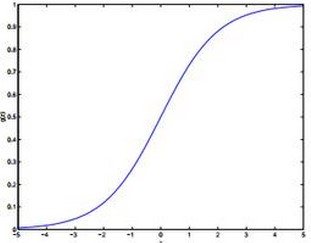Logistic回归分类器：

## 基于最优化方法的最佳回归系数确定

Sigmoid函数的输入记为z，由下面公式得出：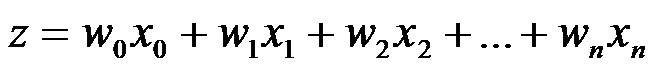## 梯度上升法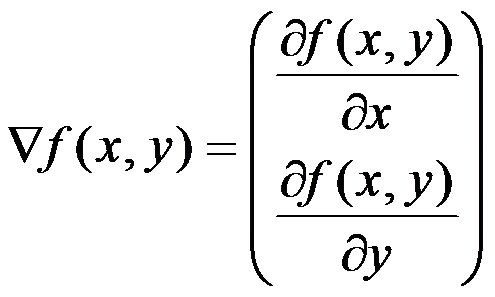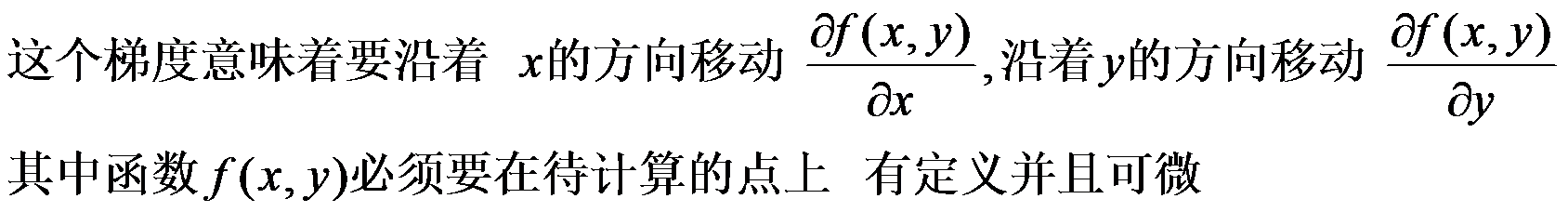## Matlab 实现


function weight = gradAscent
%%
clc
close all
clear
%%

[row , col] = size(data);
dataMat = data(:,1:col-1);
dataMat = [ones(row,1) dataMat] ;
labelMat = data(:,col);
alpha = 0.001;
maxCycle = 500;
weight = ones(col,1);
for i = 1:maxCycle
h = sigmoid((dataMat * weight)');
error = (labelMat - h');
weight = weight + alpha * dataMat' * error;
end

figure
scatter(dataMat(find(labelMat(:) == 0),2),dataMat(find(labelMat(:) == 0),3),3);
hold on
scatter(dataMat(find(labelMat(:) == 1),2),dataMat(find(labelMat(:) == 1),3),5);
hold on
x = -3:0.1:3;
y = (-weight(1)-weight(2)*x)/weight(3);
plot(x,y)
hold off

end

function returnVals = sigmoid(inX)
% 注意这里的sigmoid函数要用点除
returnVals = 1.0./(1.0+exp(-inX));
end

## 效图如下：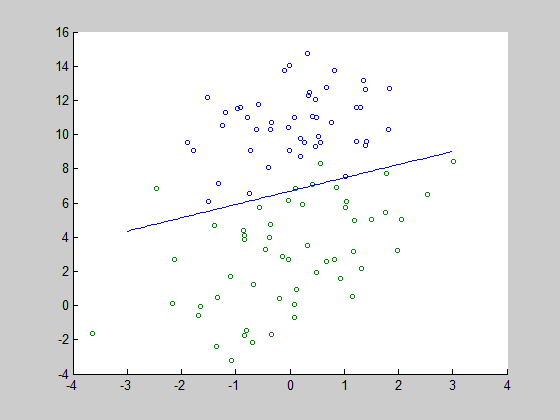## 随机梯度上升



计算该样本的梯度
使用alpha x gradient 更新回归系数值



## Matlab 代码实现


%%
%
%   Description : LogisticRegression using stocGradAsscent
%   Author : Liulongpo
%   Time：2015-4-18 10:57:25
%
%%
clc
clear
close all
%%
[row , col] = size(data);
dataMat = [ones(row,1) data(:,1:col-1)];
alpha = 0.01;
labelMat = data(:,col);
weight = ones(col,1);
for i = 1:row
h = sigmoid(dataMat(i,:)*weight);
error = labelMat(i) - h;
dataMat(i,:)
weight
weight = weight + alpha * error * dataMat(i,:)'
end

figure
scatter(dataMat(find(labelMat(:)==0),2),dataMat(find(labelMat(:)==0),3),5);
hold on
scatter(dataMat(find(labelMat(:) == 1),2),dataMat(find(labelMat(:) == 1),3),5);
hold on
x = -3:0.1:3;
y = -(weight(1)+weight(2)*x)/weight(3);
plot(x,y)
hold off

end

function returnVals = sigmoid(inX)
% 注意这里的sigmoid函数要用点除
returnVals = 1.0./(1.0+exp(-inX));
end


## 效果如下：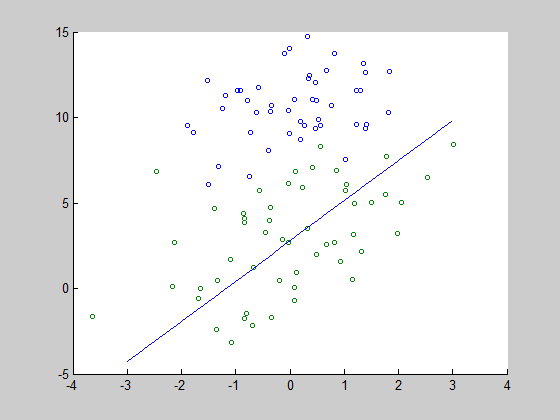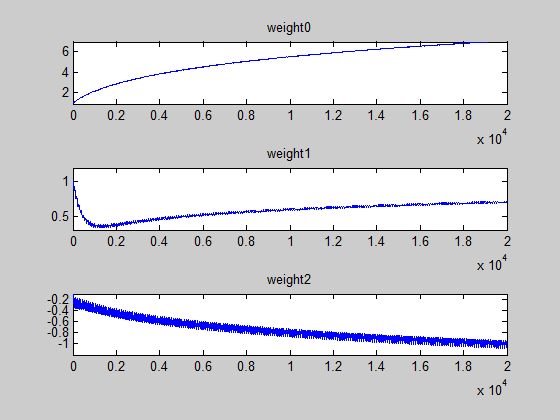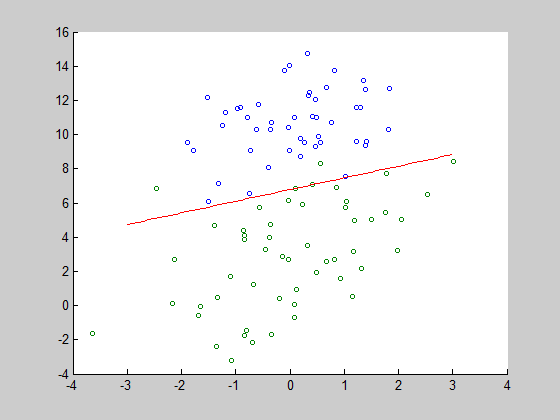## 改进的随机梯度上升算法

1,每一步调整alpha的值，也就是alpha的值是不严格下降的
2.随机采取样本来更新回归参数

## matlab代码如下：


%%
%
%   Description : LogisticRegression using stocGradAsscent
%   Author : Liulongpo
%   Time：2015-4-18 10:57:25
%
%%
clc
clear
close all
%%
[row , col] = size(data);
dataMat = [ones(row,1) data(:,1:col-1)];
%alpha = 0.01;
numIter = 20;
labelMat = data(:,col);
weightVal = zeros(3,numIter*row);
weight = ones(col,1);
j = 0;

for k = 1:numIter
randIndex = randperm(row);
for i = 1:row
% 改进点 1
alpha = 4/(1.0+i+k)+0.01;
j = j+1;
% 改进点 2
h = sigmoid(dataMat(randIndex(i),:)*weight);
% 改进点 2
error = labelMat(randIndex(i)) - h;
% 改进点 2
weight = weight + alpha * error * dataMat(randIndex(i),:)';
weightVal(1,j) = weight(1);
weightVal(2,j) = weight(2);
weightVal(3,j) = weight(3);
end
end

figure
i = 1:numIter*row;
subplot(3,1,1)
plot(i,weightVal(1,:)),title('weight0')%,axis([0 numIter*row 0.8 7])
j = 1:numIter*row;
subplot(3,1,2)
plot(j,weightVal(2,:)),title('weight1')%,axis([0 numIter*row 0.3 1.2])
k = 1:numIter*row;
subplot(3,1,3)
plot(k,weightVal(3,:)),title('weight2')%,axis([0 numIter*row -1.2 -0.1])

figure
scatter(dataMat(find(labelMat(:)==0),2),dataMat(find(labelMat(:)==0),3),5);
hold on
scatter(dataMat(find(labelMat(:) == 1),2),dataMat(find(labelMat(:) == 1),3),5);
hold on
x = -3:0.1:3;
y = -(weight(1)+weight(2)*x)/weight(3);
plot(x,y,'r')
hold off

end

function returnVals = sigmoid(inX)
% 注意这里的sigmoid函数要用点除
returnVals = 1.0./(1.0+exp(-inX));
end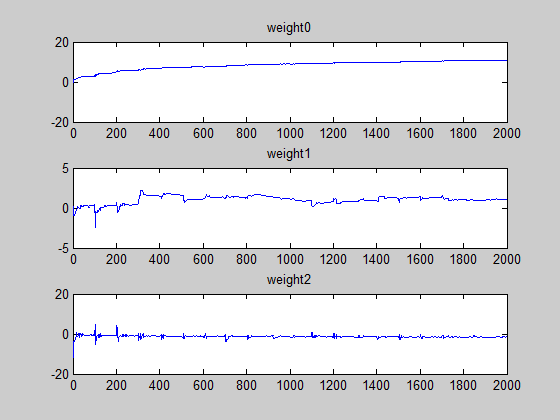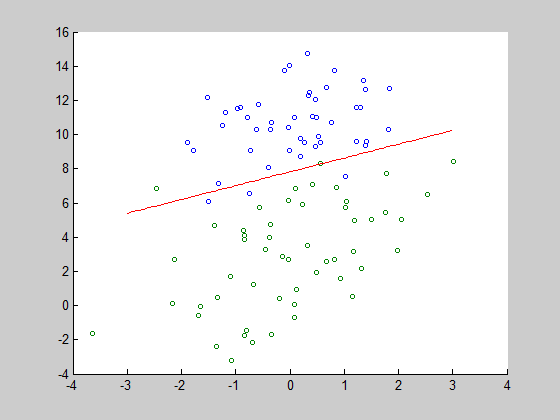## 学习率alpha的选取

h = sigmoid(dataMat(i,:)  *  weight);
error = labelMat(i) - h;
weight = weight + alpha * error * dataMat(i,:)';